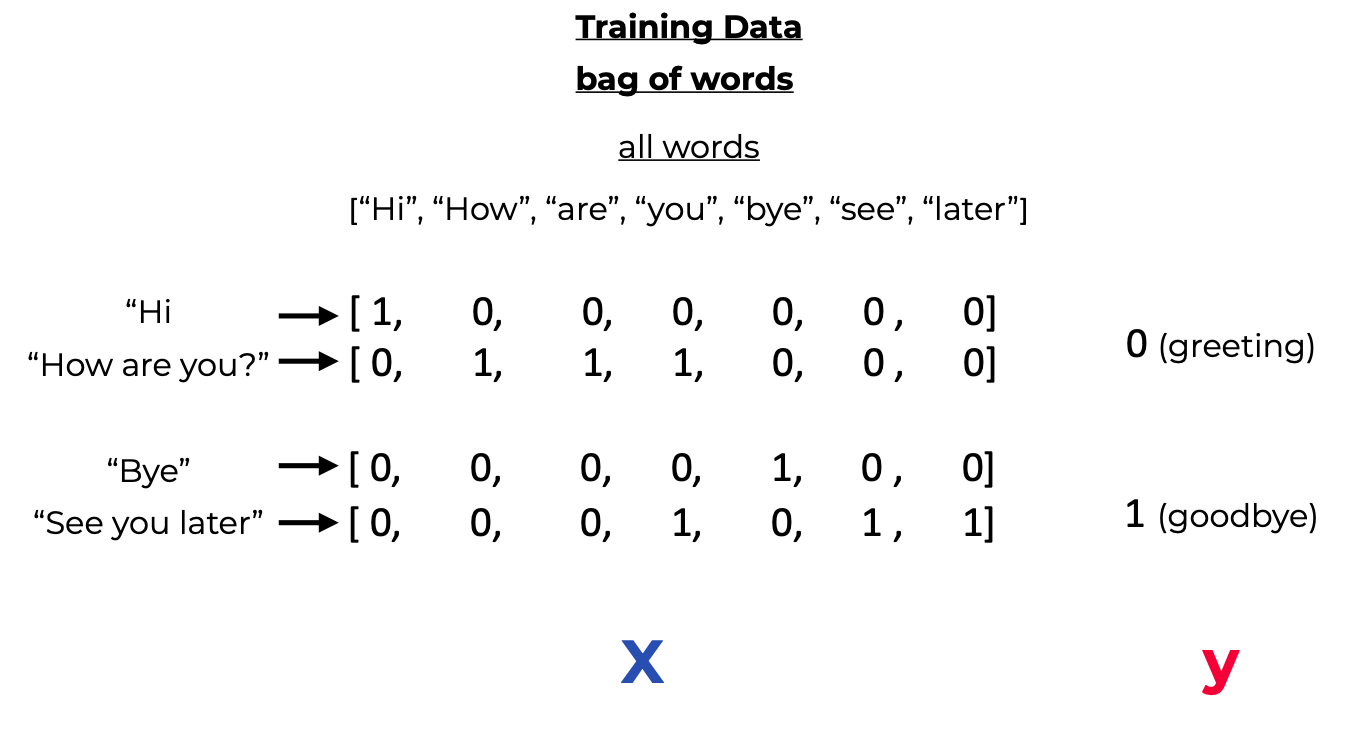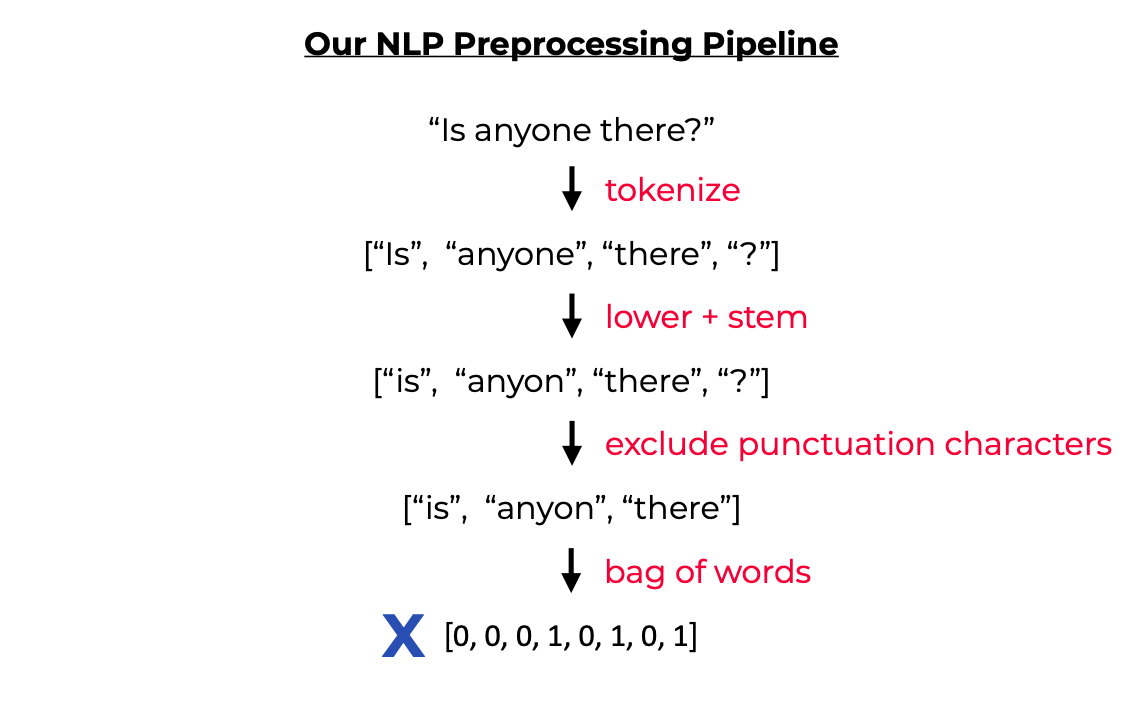# Chat Bot With PyTorch - NLP And Deep Learning

In this tutorial we build a simple chatbot in PyTorch. I will also provide an introduction to some basic Natural Language Processing (NLP) techniques.

In this tutorial we build a simple chatbot in PyTorch. I will also provide an introduction to some basic Natural Language Processing (NLP) techniques.

``````>>> Let's chat! (type 'quit' to exit)
>>> You: Hi
>>> Hi there, what can I do for you?
>>> You: What do you sell?
>>> We have coffee and tea.
``````

Here's an overview of what you will learn:

• NLP Basics: Tokenization, Stemming, Bag Of Words
• How to preprocess the data with `nltk`to feed it to your neural net
• How to implement the feed-forward neural net in Pytorch and train it
• The implementation should be easy to follow for beginners and provide a basic understanding of chatbots.
• The implementation is straightforward with a Feed Forward Neural net with 2 hidden layers.
• Customization for your own use case is super easy. Just modify `intents.json` with possible patterns and responses and re-run the training (see below for more info).

The approach is inspired by this article and ported to PyTorch: https://chatbotsmagazine.com/contextual-chat-bots-with-tensorflow-4391749d0077.

You can find the code on GitHub.

Let's start by setting up our virtual environment and installing `PyTorch` and `nltk`.

### Create an environment¶

Whatever you prefer (e.g. `conda` or `venv`)

``````mkdir myproject
\$ cd myproject
\$ python3 -m venv venv
``````

### Activate it¶

Mac / Linux:

``````. venv/bin/activate
``````
Windows:

``````venv\Scripts\activate
``````

### Install PyTorch and dependencies¶

For Installation of PyTorch see official website.

You also need `nltk`:

``````pip install nltk
``````

If you get an error during the first run, you also need to install `nltk.tokenize.punkt`: Run this once in your terminal:

``````\$ python
>>> import nltk
``````

## 2) Create Training Data¶

We need to create training data in a json file (`intents.json`). It has the following structure:

``````{
"intents": [
{
"tag": "greeting",
"patterns": [
"Hi",
"Hey",
"How are you",
"Is anyone there?",
"Hello",
"Good day"
],
"responses": [
"Hey :-)",
"Hello, thanks for visiting",
"Hi there, what can I do for you?",
"Hi there, how can I help?"
]
},
...
]
}
``````

You can customize it according to your own use case. Just define a new `tag`, possible `patterns`, and possible `responses` for the chat bot. You have to re-run the training whenever this file is modified.

## 3) NLP Basics¶

We can't just pass the input sentence as it is to our neural net. We somehow have to convert the pattern strings to numbers that the network can understand. For this we convert each sentence to a so called bag of words (bow). To do this we need to collect training words, i. e., all the words that our bot can have a look at in the training data. Based on all these words, we can then calculate the bag of word for each new sentence. A bag of words has the same size as the all words array, and each position contains a 1 if the word is avaliable in the incoming sentence, or 0 otherwise. Here's a visual example:Before we can calculate the bow, we apply two more NLP techniques: Tokenization and Stemming.

• Tokenization: Splitting a string into meaningful units (e.g. words, punctuation characters, numbers)

Example:

``````"what would you do with 1000000\$?"
[ “what”, “would”, “you”, “do”, “with”, “1000000”, “\$”, “?”]
``````
• Stemming Generate the root form of the words. It's a crude heuristic that chops of the ends off of words

Example:

``````[“organize”, “organizes”, “organizing”]
[ “organ”, “organ”, “organ”]
``````

For our labels, we sort them alphabetically and then use the index as class label. Our whole preprocessing pipeline looks like this:## 4) Implement The NLP Utils¶

For this we use the `nltk` module. NLTK (Natural Language Toolkit) is a leading platform for building Python programs to work with human language data. It provides a lot of helpful methods that we can use.

``````# nltk_utils.py
import numpy as np
import nltk
from nltk.stem.porter import PorterStemmer
stemmer = PorterStemmer()

def tokenize(sentence):
"""
split sentence into array of words/tokens
a token can be a word or punctuation character, or number
"""
return nltk.word_tokenize(sentence)

def stem(word):
"""
stemming = find the root form of the word
examples:
words = ["organize", "organizes", "organizing"]
words = [stem(w) for w in words]
-> ["organ", "organ", "organ"]
"""
return stemmer.stem(word.lower())

def bag_of_words(tokenized_sentence, words):
"""
return bag of words array:
1 for each known word that exists in the sentence, 0 otherwise
example:
sentence = ["hello", "how", "are", "you"]
words = ["hi", "hello", "I", "you", "bye", "thank", "cool"]
bog   = [  0 ,    1 ,    0 ,   1 ,    0 ,    0 ,      0]
"""
# stem each word
sentence_words = [stem(word) for word in tokenized_sentence]
# initialize bag with 0 for each word
bag = np.zeros(len(words), dtype=np.float32)
for idx, w in enumerate(words):
if w in sentence_words:
bag[idx] = 1

return bag
``````

## 5) Implement The Neural Network¶

The implementation is straightforward with a Feed Forward Neural net with 2 hidden layers:

``````# model.py
import torch
import torch.nn as nn

class NeuralNet(nn.Module):
def __init__(self, input_size, hidden_size, num_classes):
super(NeuralNet, self).__init__()
self.l1 = nn.Linear(input_size, hidden_size)
self.l2 = nn.Linear(hidden_size, hidden_size)
self.l3 = nn.Linear(hidden_size, num_classes)
self.relu = nn.ReLU()

def forward(self, x):
out = self.l1(x)
out = self.relu(out)
out = self.l2(out)
out = self.relu(out)
out = self.l3(out)
# no activation and no softmax at the end
return out
``````

## 6) Implement The Training Pipeline¶

Put everything together:

``````# train.py
import numpy as np
import random
import json

import torch
import torch.nn as nn

from nltk_utils import bag_of_words, tokenize, stem
from model import NeuralNet

with open('intents.json', 'r') as f:

all_words = []
tags = []
xy = []
# loop through each sentence in our intents patterns
for intent in intents['intents']:
tag = intent['tag']
tags.append(tag)
for pattern in intent['patterns']:
# tokenize each word in the sentence
w = tokenize(pattern)
# add to our words list
all_words.extend(w)
xy.append((w, tag))

# stem and lower each word
ignore_words = ['?', '.', '!']
all_words = [stem(w) for w in all_words if w not in ignore_words]
# remove duplicates and sort
all_words = sorted(set(all_words))
tags = sorted(set(tags))

# create training data
X_train = []
y_train = []
for (pattern_sentence, tag) in xy:
# X: bag of words for each pattern_sentence
bag = bag_of_words(pattern_sentence, all_words)
X_train.append(bag)
# y: PyTorch CrossEntropyLoss needs only class labels, not one-hot
label = tags.index(tag)
y_train.append(label)

X_train = np.array(X_train)
y_train = np.array(y_train)

# Hyper-parameters
num_epochs = 1000
batch_size = 8
learning_rate = 0.001
input_size = len(X_train)
hidden_size = 8
output_size = len(tags)

class ChatDataset(Dataset):

def __init__(self):
self.n_samples = len(X_train)
self.x_data = X_train
self.y_data = y_train

# support indexing such that dataset[i] can be used to get i-th sample
def __getitem__(self, index):
return self.x_data[index], self.y_data[index]

# we can call len(dataset) to return the size
def __len__(self):
return self.n_samples

dataset = ChatDataset()
batch_size=batch_size,
shuffle=True,
num_workers=2)

device = torch.device('cuda' if torch.cuda.is_available() else 'cpu')

model = NeuralNet(input_size, hidden_size, output_size).to(device)

# Loss and optimizer
criterion = nn.CrossEntropyLoss()

# Train the model
for epoch in range(num_epochs):
words = words.to(device)
labels = labels.to(device)

# Forward pass
outputs = model(words)
# if y would be one-hot, we must apply
# labels = torch.max(labels, 1)
loss = criterion(outputs, labels)

# Backward and optimize
loss.backward()
optimizer.step()

if (epoch+1) % 100 == 0:
print (f'Epoch [{epoch+1}/{num_epochs}], Loss: {loss.item():.4f}')

print(f'final loss: {loss.item():.4f}')

data = {
"model_state": model.state_dict(),
"input_size": input_size,
"hidden_size": hidden_size,
"output_size": output_size,
"all_words": all_words,
"tags": tags
}

FILE = "data.pth"
torch.save(data, FILE)

print(f'training complete. file saved to {FILE}')
``````

## 7) Implement The Chat¶

Load the trained model and make predictions for new sentences:

``````# chat.py
import random
import json

import torch

from model import NeuralNet
from nltk_utils import bag_of_words, tokenize

device = torch.device('cuda' if torch.cuda.is_available() else 'cpu')

with open('intents.json', 'r') as json_data:

FILE = "data.pth"

input_size = data["input_size"]
hidden_size = data["hidden_size"]
output_size = data["output_size"]
all_words = data['all_words']
tags = data['tags']
model_state = data["model_state"]

model = NeuralNet(input_size, hidden_size, output_size).to(device)
model.eval()

bot_name = "Sam"
print("Let's chat! (type 'quit' to exit)")
while True:
# sentence = "do you use credit cards?"
sentence = input("You: ")
if sentence == "quit":
break

sentence = tokenize(sentence)
X = bag_of_words(sentence, all_words)
X = X.reshape(1, X.shape)
X = torch.from_numpy(X).to(device)

output = model(X)
_, predicted = torch.max(output, dim=1)

tag = tags[predicted.item()]

probs = torch.softmax(output, dim=1)
prob = probs[predicted.item()]
if prob.item() > 0.75:
for intent in intents['intents']:
if tag == intent["tag"]:
print(f"{bot_name}: {random.choice(intent['responses'])}")
else:
print(f"{bot_name}: I do not understand...")
``````

## 8) Usage¶

Congratulations! You have implemented your chat bot! Now just run the training and start chatting 😊.

Run

``````python train.py
``````
This will dump `data.pth` file. And then run

``````python chat.py
``````

As mentioned in the beginning, you can customize it for your own needs. Just modify `intents.json` with possible patterns and responses and re-run the training.

FREE VS Code / PyCharm Extensions I Use

✅ Write cleaner code with Sourcery, instant refactoring suggestions: Link*

PySaaS: The Pure Python SaaS Starter Kit